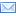#Difference Between Similar Terms and Objects

# Difference Between Sample Mean and Population MeanSample Mean vs Population Mean

“Mean” is the average of all the values in a sample. It can be calculated by adding up all the values and then dividing the sum total by the number of values in the sample.

Population Mean
When the provided list represents a statistical population, then the mean is called the population mean. It is usually denoted by the letter “µ.”

Sample Mean
When the provided list represents a statistical sample, then the mean is called the sample mean. The sample mean is denoted by “X.” It is a satisfactory estimate of the population mean.
For a sample, a population mean may be defined as:
µ = Σ x / n where;

Σ represents the sum of all the number of observations in the population;
n represents the number of observations taken for the study.

When frequency is also included in the data, then the mean may be calculated as:
µ = Σ f x / n where;

f represents the class frequency;
x represents class value;
n represents the size of the population, and
Σ represents the summation of the products “f” with “x” all over the classes.

In the same way the sample mean will be;
X = Σ x / n or
µ = Σ f x / n where “n” is the number of observations.
In a more elaborate way it may be represented as;
X = x₁ + x₂ + x₃ +…………….xn / n or
X = 1/n(x₁ + x₂ + x₃ +…………….xn ) = Σ x / n
This can be cleared with the following example:
Suppose the data has the following observations of a study.
1, 2, 2, 3, 3, 4, 5, 6, 7, 8
For these samples to take out the sample mean, we will consider several samples and consider the mean.
For 1, 2, 3, mean will be calculated as (1+ 2+3/ 3) = 2;
For 3, 4, 5, mean will be calculated as (3 +4 + 5/3) = 4;
For 4, 5, 6, 7, 8, mean will be calculated as (4 +5+6 +7 +8/5) = 6;
And for 3, 3, 4, 5, mean will be calculated as (3 + 3 +4 + 5/4) = 3.75.
Thus the total mean of these samples is (2 + 4+ 6 + 3.75/ 4) = 3.94 or approximately 4.
This value is called the sample mean.
Now for the population, the population mean can be calculated as:
1+ 2+ 2+ 3+ 3+4+5+ 6+7+ 8/10 = 4.1
Thus the sample mean is very close to the population mean. The accuracy increases with an increase in the number of samples taken.

Summary:

1.A sample mean is the mean of the statistical samples while a population mean is the mean of the total population.
2.The sample mean provides an estimate of the population mean.
3.A sample mean is more manageable data while a population mean is difficult to calculate.
4.The sample mean increases its accuracy to the population mean with the increased number of observations.

Latest posts by Nimisha Kaushik (see all)

### Search DifferenceBetween.net :

Custom Search

Help us improve. Rate this post!(4 votes, average: 3.00 out of 5)Loading...Email This Post : If you like this article or our site. Please spread the word. Share it with your friends/family.

1. it is nice explanation thank u

2. nice explanation.easy to understand.thanks.

3. thanks for this explanation. Been having issues with my ENTC class.

Please note: comment moderation is enabled and may delay your comment. There is no need to resubmit your comment.

Articles on DifferenceBetween.net are general information, and are not intended to substitute for professional advice. The information is "AS IS", "WITH ALL FAULTS". User assumes all risk of use, damage, or injury. You agree that we have no liability for any damages.